# C. No-go result forin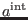We have seen in the previous two Appendixes A and B that we can always take (193) and (204) in (181). Consequently, the first-order deformation of the solution to the master equation in the interacting case stops at antighost number three(280)

where the components on the right-hand side of (205) are subject to the equations (68) and (66)-(67) for.

The piece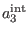as solution to equation (68) forhas the general form expressed by (75) for, with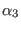from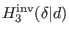. Looking at formula (76) and also at relation (81) in antighost number three and requiring thatmixes BRST generators from the BF and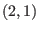sectors, we find that the most general solution to (68) forreads as8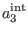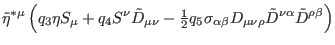(281)(282)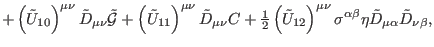(283)

where any object denoted by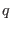represents an arbitrary, real constant. Inserting (206) in equation (66) forand using definitions (35)-(52), we can write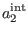(284)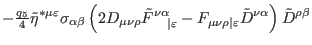(285)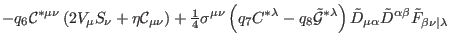(286)(287)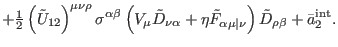(288)

The componentrepresents the solution to the homogeneous equation in antighost number two (68) for, sois a nontrivial element from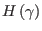of pure ghost number two and antighost number two. It is useful to decomposeas a sum between two terms(289)

withthe solution to (68) forthat ensures the consistency of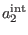in antighost number one, namely the existence ofas solution to (67) forwith respect to the terms fromcontaining the functions of the type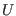or the constants denoted by, and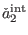the solution to (68) forthat is independently consistent in antighost number one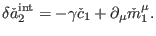(290)

Using definitions (35)-(49) and decomposition (208 ), by direct computation we obtain that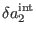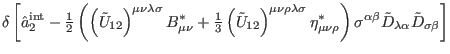(291)(292)

where we used the notations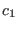(293)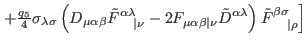(294)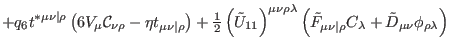(295)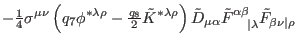(296)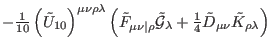(297)(298)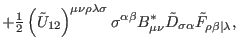(299)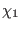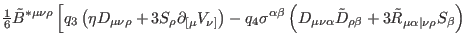(300)(301)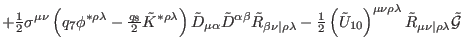(302)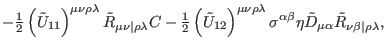(303)

andare some local currents. It is easy to see thatgiven in (212) is a nontrivial object fromin pure ghost number two, which obviously does not reduce to a full divergence. Then, since (210) requires that it is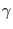-exact modulo, it must vanish, which further implies that all the functions of the typeare some real constants and all the constants denoted byvanish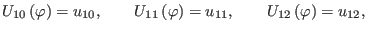(304)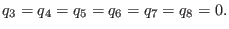(305)

Inserting conditions (213) and (214) into (206), we conclude that we conclude that we can safely take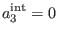(306)

in (205).

Ashkbiz Danehkar
2018-03-26